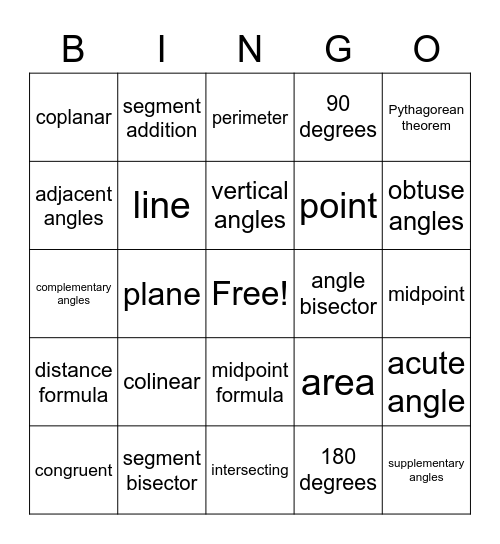Geometry VocabularyThis bingo card has a free space and 25 words: colinear, intersecting, vertical angles, congruent, Pythagorean theorem, coplanar, adjacent angles, segment addition, angle bisector, midpoint formula, point, complementary angles, area, acute angle, line, linear pairs, midpoint, perimeter, obtuse angles, plane, supplementary angles, segment bisector, distance formula, 90 degrees and 180 degrees.

Play Online